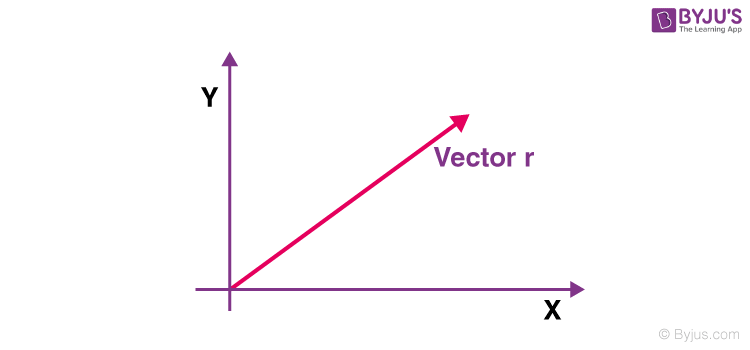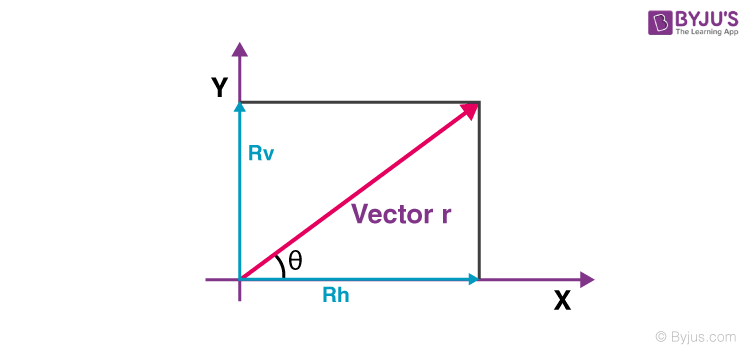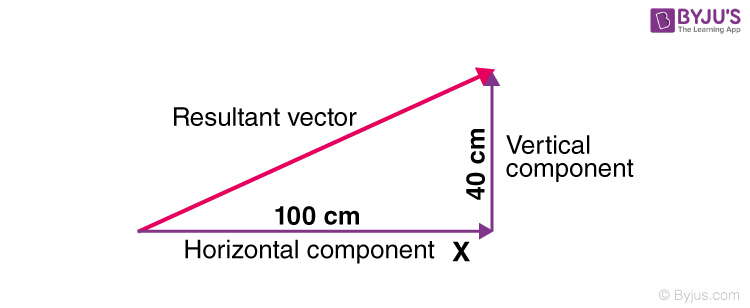# Resolution Of A Vector In A Plane - Rectangular ComponentsConsider the following vector r; the vector r can be resolved into horizontal and vertical components, these two components add up to give us the resultant vector i.e. vector r.

How do we calculate the rectangular components of a given vector?

We should know that there are two rectangular components for a vector, i.e. the horizontal component and the vertical component, the horizontal component lies on the x-axis whereas the vertical component lies on the y-axis,

Think of it this way; the horizontal component will resemble the shadow of the vector r falling on the x-axis if the light were shining from above. Similarly, the vertical component will resemble the shadow of vector r falling on the y-axis if the light were shining from the side.Now let us call the vertical component $$\overrightarrow{r_v}$$ and the horizontal vector as $$\overrightarrow{r_h}$$ and let us call the angle made by the vector $$\overrightarrow{r}$$ with the horizontal component as θ.

If we notice carefully the 3 vector $$\overrightarrow{r_v}$$ $$\overrightarrow{r_h}$$ and $$\overrightarrow{r}$$ form the 3 sides of a right angled triangle, so from trigonometry we can say that,

| $$\overrightarrow{r_h}$$ | = | $$\overrightarrow{r} | cos \theta$$

The reason is for the angle $$\theta$$ r is the hypotenuse and ris the adjacent side, so adj/hyp = cosine of the angle, so from this rule we can find the magnitude of the horizontal vector given that we know the magnitude of the vector r and the angle it makes with the horizontal vector.

Similarly, the magnitude of the vertical component can be found using the sine function because the vertical component resembles the opposite side of the triangle and opp/hyp = sine of the angle, thereby the magnitude of the vertical component is given by,

| $$\overrightarrow{r_v}$$ | = | $$\overrightarrow{r} | sin \theta$$

Now that we know how to get the magnitude of the rectangular components of the two vectors how do we find out the direction and the magnitude of the resultant vector if its horizontal and vertical components are given, this could be done easily with a graphical method,

Imagine we have the horizontal component of magnitude 100 Newtons and a vertical component of magnitude 40 Newtons then we can draw a right-angled triangle with the given data. By plotting the lengths of the vectors proportional to their magnitude. i.e.Now the resultant vector could be drawn as the hypotenuse, and the length of the vector gives us the magnitude of the resultant vector as well as its direction.